• 目录一、插值与图像缩放二、最近邻插值1、原理2、代码实现三、双线性插值1、原理2、代码实现 一、插值与图像缩放   首先举个例子说明插值过程，先看看matlab的插值函数 interp() 吧： x = -2 : 1 : 2; y = -2 : 1 ...
• 使用临近插值法和双线性插值法进行图像处理，将彩色图像缩放之后仍输出彩色图像
• Write a function Reduce / Expand that has an image as an input and the output should be re-sampled copy of half / twice the width and height of the input image.
• MATLAB图像缩放,matlab实现图像缩放,matlab源码
• % matlab库函数，调用imresize可以重新生成不同尺寸的图片   cubic_factor函数 % 距离系数计算公式 % ┏ 1 - 2 *Abs(x)^ 2 +Abs(x)^ 3 　, 0 (x)< 1 % S(x)=｛ 4 - 8 *Abs(x)+ 5 *...
http://www.cnblogs.com/xy-kidult/p/3404035.html

主要有两个代码，一个是主代码，包括注释，另一个是cubic_factor函数，用于计算距离系数的。clear;
close all;
clc;

CONST=50;
bwimg=im2bw(A);
resize_img=[];
[srcWidth srcHeight]=size(bwimg);

% %
% 以下算法思路参考http://blog.csdn.net/ArtX/article/details/1540539和http://blog.csdn.net/qiqi5521/article/details/2207562这两篇博客，谢谢博主。
% % 最近邻
% % 思想是根据 srcWidth/dstWidth = srcX/dstY，最后再对srcX取整
% % 缺点是精度不够，严重失真
%
% dstWidth=srcWidth+CONST*2;
% dstHeight=srcHeight+CONST*2;
% resize_img=zeros(dstWidth,dstHeight);
%
% % 像素变化公式计算公式
% for i=1:dstWidth
%     for j=1:dstHeight
%     src_i=i*(srcWidth/dstWidth);
%     src_j=j*(srcHeight/dstHeight);
%     resize_img(i,j)=bwimg(round(src_i),round(src_j)); % round四舍五入
%     end
% end
%
% figure,imshow(bwimg);
% figure,imshow(resize_img)

% % 双线性内插值算法
% % 聪明的方法
% % 最近邻法中根据 srcWidth/dstWidth = srcX/dstY，可以倒退出一个srcX的坐标值，但是这个值是浮点数，
% % 这个浮点数提供了一些信息，它离它在原始图像四周的像素值的关系，根据这个关系，计算一个更接近的dstX，
% % 然后再填充目标图像
% % 公式：
% % f(i+u,j+v)=(1-u)(1-v)f(i,j)+(1-u)vf(i,j+1)+u(1-v)f(i+1,j)+uvf(i+1,j+1)
% % i,j 为浮点坐标的整数部分； u,v为浮点坐标的小数部分
% % 优点：缩放后图像质量高
% % 缺点： 计算量大，双线性插值具有低通滤波器的性质，使高频分量受损，图像轮廓在一定程度上变模糊
%
% dstWidth=srcWidth+CONST*2;
% dstHeight=srcHeight+CONST*2;
% resize_img=zeros(dstWidth,dstHeight);
%
% % 像素变化公式计算公式
% for i=1:dstWidth-1
%     for j=1:dstHeight-1
%     src_i=i*(srcWidth/dstWidth);
%     src_j=j*(srcHeight/dstHeight);
%     src_ii=fix(src_i);
%     src_iu=src_i - src_ii; % none fix part
%     src_jj=fix(src_j);
%     src_jv=src_j - src_jj;
%     if src_ii == 0
%         src_ii=src_ii+1;
%     end
%     if src_jj ==0
%         src_jj=src_jj+1;
%     end
%     resize_img(i,j)=(1-src_iu)*(1-src_jv)*bwimg(src_ii,src_jj)+(1-src_iu)*src_jv*bwimg(src_ii,src_jj+1)+src_iu*(1-src_jv)*bwimg(src_ii+1,src_jj)...
%         +src_iu*src_jv*bwimg(src_ii+1,src_jj+1);
%     end
% end
% figure,imshow(bwimg);
% figure,imshow(resize_img)

% 三次卷积法
% 三次卷积法计算精度高，但计算亮大，思路是根据一个浮点坐标(i+u,j+v)周围的16个邻点，由下列公式得出目的像素值f(i+u,j+v)：
%
% 　　　　f(i+u,j+v) = [A] * [B] * [C]
%
% [A]=[ S(u + 1)　S(u + 0)　S(u - 1)　S(u - 2) ]
%
% 　　┏ f(i-1, j-1)　f(i-1, j+0)　f(i-1, j+1)　f(i-1, j+2) ┓
% [B]=┃ f(i+0, j-1)　f(i+0, j+0)　f(i+0, j+1)　f(i+0, j+2) ┃
% 　　┃ f(i+1, j-1)　f(i+1, j+0)　f(i+1, j+1)　f(i+1, j+2) ┃
% 　　┗ f(i+2, j-1)　f(i+2, j+0)　f(i+2, j+1)　f(i+2, j+2) ┛
%
% 　　┏ S(v + 1) ┓
% [C]=┃ S(v + 0) ┃
% 　　┃ S(v - 1) ┃
% 　　┗ S(v - 2) ┛
%
% 　　 ┏ 1-2*Abs(x)^2+Abs(x)^3　　　　　 , 0<=Abs(x)<1
% S(x)=｛ 4-8*Abs(x)+5*Abs(x)^2-Abs(x)^3　, 1<=Abs(x)<2
% 　　 ┗ 0　　　　　　　　　　　　　　　 , Abs(x)>=2
% 有人说，S(x)是对 Sin(x*Pi)/x
% 的逼近（Pi是圆周率——π），通过极限计算，可以知道Sin(x*Pi)/x的范围在0~pi之间，无法理解为什么要这样设置S(x）
% 但上述求A、B、C的公式还是可以理解，根据双线性插值的思想，也就是根据浮点数在原始坐标中与邻近像素的距离远近来决定原始坐标在目的坐标中所占的比重

dstWidth=srcWidth-CONST*2;
dstHeight=srcHeight-CONST*2;
resize_img=zeros(dstWidth,dstHeight);
A=[];B=[];C=[];

% 像素变化公式计算公式
for i=3:dstWidth-2
for j=3:dstHeight-2
src_i=i*(srcWidth/dstWidth);
src_j=j*(srcHeight/dstHeight);
src_ii=fix(src_i); % 整数部分
src_iu=src_i - src_ii; % 小数部分
src_jj=fix(src_j);
src_jv=src_j - src_jj;

A=[ cubic_factor(src_iu+1) cubic_factor(src_iu) cubic_factor(src_iu-1) cubic_factor(src_iu-2) ];
C=[ cubic_factor(src_jv+1); cubic_factor(src_jv); cubic_factor(src_jv-1); cubic_factor(src_jv-2) ];
B=[ bwimg(src_ii -1,src_jj -1) bwimg(src_ii-1,src_jj) bwimg(src_ii-1,src_jj+1) bwimg(src_ii-1,src_jj+2);...
bwimg(src_ii,src_jj-1) bwimg(src_ii,src_jj) bwimg(src_ii,src_jj+1) bwimg(src_ii,src_jj+2); ...
bwimg(src_ii+1,src_jj-1) bwimg(src_ii+1,src_jj) bwimg(src_ii+1,src_jj+1) bwimg(src_ii+1,src_jj+2); ...
bwimg(src_ii+2,src_jj-1) bwimg(src_ii+2,src_jj) bwimg(src_ii+2,src_jj+1) bwimg(src_ii+2,src_jj+2) ];

resize_img(i,j)=A*B*C;

end
end

figure,imshow(bwimg);
figure,imshow(resize_img)

% dstimg=imresize(srcimg,4,'bicubic'); % matlab库函数，调用imresize可以重新生成不同尺寸的图片cubic_factor函数% 距离系数计算公式
% 　　 ┏ 1-2*Abs(x)^2+Abs(x)^3　　　　　 , 0<=Abs(x)<1
% S(x)=｛ 4-8*Abs(x)+5*Abs(x)^2-Abs(x)^3　, 1<=Abs(x)<2
% 　　 ┗ 0　　　　　　　　　　　　　　　 , Abs(x)>=2
function S=cubic_factor(x)

if 0<=abs(x)<1
S=1-2.*abs(x).^2+abs(x).^3;
elseif 1<=abs(x)<2
S=4-8.*abs(x)+5.*abs(x).^2-abs(x).^3;
else
S=0;
end上面代码有一个问题，”最右边和最下边的像素实际上并没有参与计算“，似乎是由于最初的中心点选择方法引起的，网上有一个人提出了解决方案(http://handspeaker.iteye.com/blog/1545126)，看了一下，大概了解他的想法，试验之后，没有效果，可能我理解错了，下次再来解决这个问题并修改代码吧。

展开全文算法
• 包含最近邻插值、双线性插值和三次插值的matlab代码，自己已经测试过，使用的时候注意将图片转换为位图，否则会出现错误。
• 自编Matlab函数实现图像缩放 自编Matlab函数实现图像缩放 自编Matlab函数实现图像缩放 自编Matlab函数实现图像缩放 自编Matlab函数实现图像缩放
• ## matlab实现图片缩放

千次阅读 2019-07-07 15:38:37
zoomNum为缩放系数 height和width的命名可能反了，不要在意这些细节… I=imread('tissue.png'); figure(1); imshow(I) zoomNum=0.5; [fWidth fHeight]=size(I); fHeight=fHeight/3; cWidth=floor(fWidth*...
利用双线性插值实现 zoomNum为缩放系数 height和width的命名可能反了，不要在意这些细节… 一开始用subplot输出两幅图片，明明已经得到一个更大的矩阵了但就是死活看不出效果，后来求助于助教才知道subplot会让它们输出大小一致（吐血）…
    I=imread('tissue.png');
figure(1);
imshow(I)
zoomNum=0.5;
[fWidth fHeight]=size(I);
fHeight=fHeight/3;
cWidth=floor(fWidth*zoomNum);
cHeight=floor(fHeight*zoomNum);
res=zeros(cWidth,cHeight,3);
%四个顶点
res(1,1,:)=I(1,1,:);
res(1,cHeight,:)=I(1,fHeight,:);
res(cWidth,1,:)=I(fWidth,1,:);
res(cWidth,cHeight,:)=I(fWidth,fHeight,:);
%四条边界
for dstX=2:cWidth-1
srcX=dstX/zoomNum;
i=floor(srcX);u=srcX-i;
res(dstX,1,:)=u*I(i,1,:)+(1-u)*I(i+1,1,:);
res(dstX,cHeight,:)=u*I(i,fHeight,:)+(1-u)*I(i+1,fHeight,:);
end
for dstY=2:cHeight-1
srcY=dstY/zoomNum;
i=floor(srcY);u=srcY-i;
res(cWidth,dstY,:)=u*I(fWidth,i,:)+(1-u)*I(fWidth,i+1,:);
res(1,dstY,:)=u*I(1,i,:)+(1-u)*I(1,i+1,:);
end
%中间
for dstX=2:cWidth-1
for dstY=2:cHeight-1
srcX=dstX/zoomNum;
srcY=dstY/zoomNum;
i=floor(srcX);u=srcX-i;
j=floor(srcY);v=srcY-j;
res(dstX,dstY,:)=(1-u)*(1-v)*I(i,j,:)+(1-u)*v*I(i,j+1,:)+u*(1-v)*I(i+1,j,:)+u*v*I(i+1,j+1,:);
end
end
figure(2);
imshow(uint8(res));

展开全文• 利用matlab进行图像的一些操作处理 缩放和旋转功能的实现
• 以灰度图像circuit.tif为例，利用Matlab图像处理工具箱中的imresize函数对图像进行比例缩放变换。要求：创建4个figure窗口（不可以用subplot，显示不出来放大效果），分别用于显示原始图像、等比例放大1.5倍后的图像...
以灰度图像circuit.tif为例，利用Matlab图像处理工具箱中的imresize函数对图像进行比例缩放变换。要求：创建4个figure窗口（不可以用subplot，显示不出来放大效果），分别用于显示原始图像、等比例放大1.5倍后的图像、等比例缩小0.5倍后的图像、缩放为高190宽400的图像（实现不等比例缩放）。并保存缩放后的所有图像文件到当前目录中。
I=imread('circuit.tif');
F=imresize(I,1.5,'nearest');
imwrite(F,'circuitFangda1_5.tif');
S=imresize(I,0.5,'nearest');
imwrite(S,'circuitSuoxiao0_5.tif');
J=imresize(I, [190,400],'nearest');
imwrite(J,'circuitbudengbi190_400.tif');
figure(1);
imshow(I);
figure(2);
imshow(F);
figure(3);
imshow(S);
figure(4);
imshow(J);


展开全文• matlab开发-使用交叉像素插值进行图像缩放。使用跨像素插值算法放大NxM图像
• ## Matlab——图像缩放（插值法）

万次阅读 多人点赞 2018-07-30 15:49:36
用双线性内插法实现位深度为8的灰度图像缩放。   思路：  输入原图像以及缩放图像的像素要求（宽度*高度），处理后输出新图像。  我是用matlab来实现scale(input_img,scale_size)函数的，输入图像路径...
实验内容：
用双线性内插法实现位深度为8的灰度图像的缩放。

思路：
输入原图像以及缩放后图像的像素要求（宽度*高度），处理后输出新图像。
我是用matlab来实现scale(input_img,scale_size)函数的，输入图像路径以及要求实现的宽度scale_w和高度值scale_h即可。通过matlab把原图像转化为img矩阵，这样矩阵img(a, b)元素的值就是原图像高度为a，宽度为b处的像素值。
设处理前后图像的宽度和高度分别为w、h，scale_w、scale_h。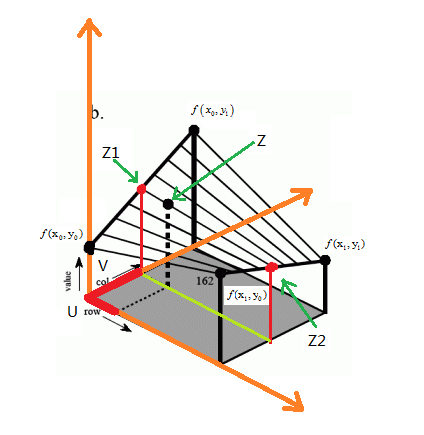如上图所示，row对应的是高度，设为x轴；col对应的是宽度，设为y轴；value对应的是灰度值，设为z轴。

通过缩放比例，可以求得新图像B在(i,j)处对应原图像的点为(x,y)（其中x= i*h/scale_h，y= j*w/scale_w）。
但是这两个值可能为浮点数，而像素中的位置是整数，所以这个点在原图像中对应的可能是“虚”点。所以，我们需
要根据找该“虚”点周围的四个点来进行双线性插值得到新图像的灰度值。我们应该取的是(x,y)邻近的四个像素
(x0,y0) 、(x0,y1) 、(x1,y0) 、(x1,y1)，它们对应的灰度值为f(x0,y0) 、f(x0,y1)、 f(x1,y0)、 f(x1,y1)。

先对x方向进行插值，再对y方向进行插值，计算如下：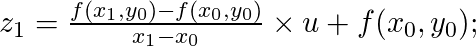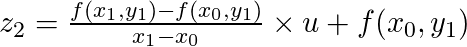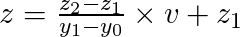反过来，先对y方向：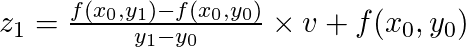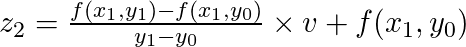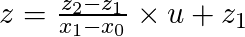显然，邻近像素的x1-x0 = y1-y0 = 1     整理式子得到：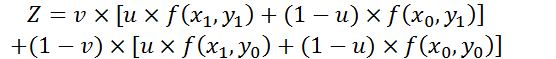这里u、v分别是x、y的小数部分，即u = x - x0; v = y - y0
要注意的是：上述公式img(a,b)中的a和b不能小于1，所以当x和y小于1时需要将其重新赋值为1。

代码：
function output_img = scale(input_img, scale_size)
%Input - input_img is a two-dimensional matrices storing image
%      - scale_size is a tuple of [width, height] defining the spatial resolution of output
%Output - output_img is the same as input_img

[h,w] = size(img); %获取行和列，即原图的高度和宽度

scale_w = scale_size(1); %根据输入获得缩放后的新宽度
scale_h = scale_size(2); %根据输入获得缩放后的新高度
output_img = zeros(scale_h, scale_w); %初始化

for i = 1 : scale_h         %缩放后的图像的（i,j）位置对应原图的（x,y）
for j = 1 : scale_w
x = i * h / scale_h;
y = j * w / scale_w;
u = x - floor(x);
v = y - floor(y); %取小数部分

if x < 1           %边界处理
x = 1;
end

if y < 1
y = 1;
end

%用原图的四个真实像素点来双线性插值获得“虚”像素的像素值
output_img(i, j) = img(floor(x), floor(y)) * (1-u) * (1-v) + ...
img(floor(x), ceil(y)) * (1-u) * v + ...
img(ceil(x), floor(y)) * u * (1-v) + ...
img(ceil(x), ceil(y)) * u * v;
end
end

imwrite(uint8(output_img), '../output_img.png'); %保存处理后的图像
imshow(input_img); %显示原图
figure,imshow(uint8(output_img)) %显示处理后的图像

运行时若图片在代码上一级目录，则输入scale('../a.png', [450,300]);

效果图：
原图为384*256：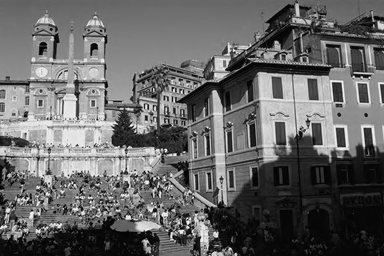缩小成192*128：放大成450*300：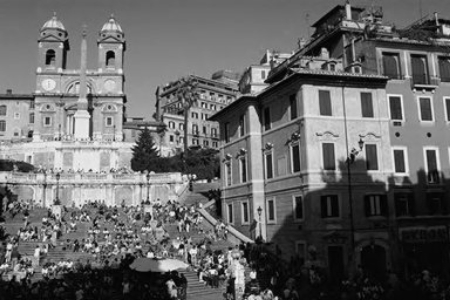缩放成500*200：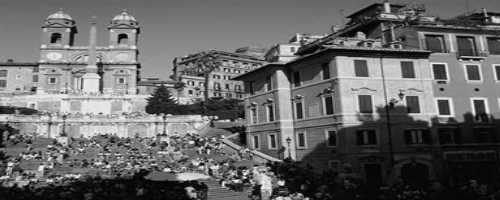//
转载：https://blog.csdn.net/Stella_Chan/article/details/78025020?locationNum=5&fps=1
展开全文插值法
• Matlab图像缩放 Matlab实现函数代码如下： clc I=rgb2gray(imread('Campus-scenery2sk.JPG')); figure,imshow(I); title('原图'); reduceI=imresize(I,0.5); %原图像I缩小0.5倍 figure,imshow(reduceI); title('...
• 经典图像/视频缩放算法原理及MATLAB\Simulink实现1 图像/视频缩放介绍2 图像缩放的经典算法2.1 最近邻插值法2.2 双线性插值法3 图像缩放算法的MATLAB实现3.1 最近邻算法实现3.2 双线性插值算法实现4 视频无极缩放的...
• % 图像缩放函数 % f为输入RGB图像，s为输出RGB图像 % scare表示缩放因子
• %读一幅图像 j=imrotate(i,30%图像旋转30度 k=imresize(i,2%图像放大两倍 t=imresize(i,2'bilinear%采用双线性插值法进行放大两倍 m=imresize(i,0.8%图像缩小到0.8倍 p=translate(strel(1, [25 25]%图像平移 img=...
• matlab实现图像缩放 缩放同样是仿射变换的一种特例，它接受水平和垂直两个方向的缩放值。 matlab 实现水平，垂直，水平垂直缩放： clear all close all clc img = imread('test.jpg'); %读取输入图片的...
• ## MATLAB:图片缩放

千次阅读 2018-10-21 17:31:33
将指定文件夹中的所有图片一次缩小后保存，名字与原来的名字相同。 核心语句： S=imresize(rgb,1/6,'nearest'); 整体代码： file_path = 'F:\2018\'; % 图像文件夹路径 img_path_list = dir(strcat(file_path,'*....
• 本代码是matlab图像缩放和旋转代码，里面使用了最近领域实现和线性插值法实现算法实现图像缩放和旋转
• 基于matlab图像放大代码，操作简单速度快。
• t=imread('图像处理.png'); [m,n,dep]=size(t); rm=3630; rn=2355; rt=zeros(rm,rn,dep); for i=1:rm for j=1:rn x=i*m/rm; y=j*n/rn; u=x-floor(x); v=y-floor(y); if x<1 x=1; end if y<1 y=1; end rt(i,j,:...计算机视觉
• %% ----------------------双线性插值法缩放矩阵或图像--------------------------- % Input： % I：图像文件名或矩阵（整数值（0~255）） % zmf：缩放因子，即缩放的倍数 % Output： % 缩放后的图像矩阵 ZI % Usage...
• 可以通过给定的正因子缩放给定的图像。 复制nearest_neighbor_zoom.m 文件和应该放大到您的工作目录的图像文件。 如果图像文件的名称是 image.jpg 并且需要的缩放系数是 2.23， 在命令窗口上运行以下命令。 Nearest_...
• 一般来讲，图像进行缩放时，原始像素间的相对位置会发生改变，产生几何畸变。比如放大1.6倍，原像素就会向新像素进行映射，此时则会产生小数位的像素，然而像素是不能处理小数位的，所以需要通过插值法计算出新的...
• 参考：https://www.cnblogs.com/zangyu/p/5395504.html对图像进行固定大小的缩放clear; clc; ％清除以前的数据 folderName = dir('train'); ％显示train文件夹下的文件和文件夹 for i = 1 : length(folderNa...
• MATLAB图像分辨率的增强与图像缩放循环重写，本质上是矩阵的数据刷新，重新布局计算。 源码整理如下： % 关闭所有窗口，清理变量，清理屏幕 close all;clear all;clc AI = imread('dfgdg.jpg'); imshow(AI)%显示原图...计算机视觉 机器学习...

# matlab图像缩放matlab 订阅